## Temperature and Heat

Temperature sensors, thermocouples, thermistors, and power transistor heat design

### Silicon Temperature Sensors

Temperature and heat play a large role in electronics. It's heat that makes PN junctions and transistors work, and gives us the relation I = Io exp (qV/kT) for the current across a forward-biased PN junction. T is the absolute temperature, q the charge on the electron, and k Boltzmann's constant (the gas constant per molecule). kT/q = 25 mV at room temperature, and is called the thermal voltage. 3kT/2 is the average kinetic energy of a free molecule, and kT the average energy of a harmonic oscillator at temperature T. The absolute temperature T is usually measured in kelvin, K, and 0°C is 273.15K. Temperature is actually not a fundamental concept, just the rate of change of energy with entropy of a system, but is important because it specifies thermal equilibrium, and the direction of heat flow, on which our personal comfort also depends. The unit of temperature is arbitrary, as is zero point of temperature scales linearly related to absolute temperature, such as Celsius, Fahrenheit or Réaumur. None is any more "metric" than another, but the Celsius scale is the most widely used, and its degree is the same as the Kelvin degree. The Fahrenheit degree is 5/9 of the Celsius degree, and is 32 when Celsius is zero. All these things are familiar.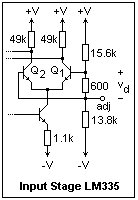It is not surprising that PN junctions can be used for thermometry. The differential voltage vd between the bases of a differential amplifier is related to the currents in the transistors by vd = (kT/q) ln (I1/I2). If the ratio of the currents can be held constant, then this voltage is proportional to the absolute temperature, and gives a linear thermometer. This principle is used in the LM335 temperature sensor, whose input stage is shown at the right. A voltage divider makes vd exactly 1/50 of the voltage across the device. The difference in the collector currents of the two transistors in the differential amplifier stage is fed back to a circuit that adjusts the voltage across the device until a constant ratio of collector currents results. Since this voltage is proportional to vd, it is proportional to the absolute temperature. The current ratio is selected to make the proportionality constant 10 mV/K.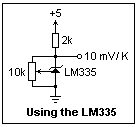The LM335 is used as in the diagram on the left. The 2k resistor programs the current to about 1 mA, since the voltage across the LM335 is about 3 V at room temperature. The 10k potentiometer calibrates the device to exactly 10 mV/K by a single adjustment, which serves for all temperatures, since the output is linear and proportional to absolute temperature. The potentiometer can usually be omitted, since even a random device is fairly well calibrated, and will be in error by only a degree or two. The LM335 is specified for -40 to 100 °C. Its cousin LM235 for -40 to 125 °C, and the LM135 over the full range of -55 to 150 °C. Each of the types can be used intermittently at higher temperatures, up to 200 °C for the LM135, but the life is reduced. At lower temperatures, of course, silicon stops being a semiconductor. The greatest restriction on semiconductor temperature sensors is the limited temperature range, but this range includes most environmental temperatures, so it is very useful. The best thing about them is that they are linear, and no calibration curves are necessary.If you want a voltage proportional to Celsius temperatures, you must subtract a constant 2.7315 V from the output of the sensor. This can be done with op-amp voltage references, but is a bother. The easy way is to use an LM35 Celsius temperature sensor, that includes all of this internally. The LM35 comes in the same TO-92 package as the LM335, but the connections are quite different, as shown in the diagram on the right. The LM35 needs no programming resistor, and is returned to ground. The output may be pulled below ground by a resistor that sinks 50 μA from the output, if you want to measure negative temperatures. The circuit is dead simple, as shown at the left.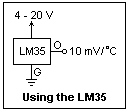These sensors are very well calibrated. A random example gave 0.277 V output, or 27.7 °C, when a good mercury thermometer read 27.5 °C, and this is much closer than required for government work. The LM35 is an excellent choice for a thermometer. There is a related Fahrenheit sensor, the LM34.

The speed of response of a sensor depends on how rapidly its temperature agrees with that of its surroundings, whose temperature is desired. In still air, the TO-92 package approaches 90% of its final value in 2 minutes, and is practically in equilibrium after 4 minutes. In a stirred oil bath, these times are reduced to 2 seconds and 4 seconds, respectively. The thermal time constants quoted are 80s in still air, 10s in 100 ft/min air, and 1s in stirred oil. These times will be affected by any covering of the TO-92 to protect it from liquids. To measure the temperature of a solid, the package can be cemented to the surface to ensure good thermal contact. The usual slip-on heat sinks can also reduce the equilibration time. In many cases, a desire to measure temperature to fractions of a degree is relatively useless, since there may be no uniformity or equilibration in the surroundings.

### Thermocouples

Suppose two different conducting wires are brought into contact at the ends, making a closed loop. Charge carriers can move back and forth between the two conductors at each contact. Usually the rates in the two directions are not the same, so one conductor loses electrons and becomes positive, and the other gains electrons and becomes negative. The difference in potential adjusts the rates so that they are equal in equilibrium. The result is a contact potential. The minute you try to measure this contact potential, you are frustrated, because you introduce other contacts. All the conductors assume their potentials, but no current flows in equilibrium. However, if the two contacts are at different temperatures, the corresponding charge carrier rates of flow are usually different, so the contact potential is different, and a current flows. An electron picks up energy at the end with greater contact potential, and releases less energy at the end with the lesser contact potential; the difference appears as resistive heat. This is a kind of heat engine, which is called the Peltier effect, while the difference in potentials is called the Seebeck effect. The Seebeck voltage can be used to measure temperature. These thermoelectric effects occur whenever two different conductors are joined, not just when we want to measure temperature, and can be the source of strange behavior in some circuits.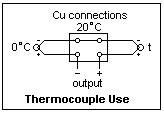A thermocouple is a junction between two dissimilar metals, usually welded or brazed. If you take two such thermocouples, and connect the same metals on one side, and measure the voltage between the same metals on the other side, you will find the Seebeck voltage if the two junctions are at different temperatures. The junctions with the copper measurement wires exactly cancel out, if they are at the same temperature. The circuit is shown in the diagram at the right. One junction is usually held at 0 °C in an ice-water bath, and is called the cold junction. The other is the hot junction.

The combinations generally used are standardized. A chromel (90 Ni, 10 Cr) - alumel (96 Ni, 2 Mn, 2 Al) couple is called type K, and gives about 40.28 μV/K at room temperature, and is useful up to about 1370 °C. An iron - constantan (55 Cu, 45 Ni) couple is called type J, gives about 51.45 μV/K, and is good up to 760 °C. A copper - constantan couple is called type T, gives 40.28 μV/K, and is good to 400 °C. Sometimes seen is the type S couple, platinum - 90 Pt 10 Rh, good up to 1750 °C, but giving only 5.88 μV/K. The voltage change with temperature is not linear, but tables are available to correlate voltage and temperature. There are many variations in the alloys, and the numbers in the references do not always agree exactly. For rough estimation, 40 μV/K can be used for types K and T thermocouples, which gives 1.00 mV at 25 °C.

It is somewhat inconvenient to mess with an ice-water bath, and the bath must be stirred for good accuracy as well. The cold junction can be replaced by a cold junction compensation circuit that furnishes the same change in voltage that the couple itself would. If the ambient temperature is above 0 °C, the extra voltage is supplied to make the output the same as if an actual cold junction was used. At 0 °C, the circuit must cancel the emf from the junctions of the thermocouple metals to copper, to give a zero output. At other temperatures, it should give the same mV difference as a cold junction.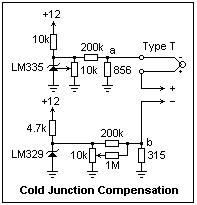A circuit that will do this is shown at the left, with values for a type T couple. This is not the most convenient circuit, but it shows the principle clearly. The upper section uses an LM335 to make a voltage that increases at the same rate as the type T thermocouple voltage, about 40 μV/K. The voltage divider resistors (200k and 856, 200k and 315) should be 1% resistors. The lower section uses an LM329 6.9V reference to provide a constant voltage equal to the ouput of the LM335 section at 0 °C., plus the thermovoltage, about 11 mV. My LM329 gave 6.97V (specs give 6.7 to 7.2 V). Whatever the voltage, it will not change with temperature (no more than 20 ppm/K for the B version).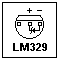Then, when the hot junction is also at 0 °C, the output is zero, as it should be. The zero adjustment on the LM329 side is very useful.When the ambient temperature increases, the equivalent voltage from that temperature to zero is added in, just as if a cold junction were present. All the copper connections should be at the same temperature to avoid unwanted thermovoltages. Simply to test the circuit, the resistors need not be exact values. 820 and 300 ohms give reasonable results.

I tested the circuits with what I believed to be a type K thermocouple. Putting the thermocouple in ice tea, the output was adjusted to 0 mV with the 10k pot. The thermocouple by itself gave -1.4 mV in ice tea when measured by the DMM (some references seem to think this will be zero, but it's not). When the thermocouple was brought to room temperature (which was about 25 °C, since it was summer), the DMM showed 1.0 mV. This was on the edge of the meter's sensitivity, so the measurement was rough, showing only that the circuit was probably working. If you do not get reasonable results, interchange the thermocouple leads. They look the same, but there are two possibilities, and Murphy's Law says that you will initially make the wrong assumption. Thermocouples can be purchased from Omega Corporation in packages of four. Working with this circuit will show you how cold junction compensation works. Next winter, I shall take it outdoors to actually test the compensation.

Arrays of thermocouples can be used for heating or cooling, taking advantage of the Peltier heat. These devices require large currents at low voltages, and are not very efficient because of heat conduction between the hot and cold sides, which are close together and connected by metal. Nevertheless, these coolers (or heaters) are useful in unusual applications. Thermocouples can also be used to generate electricity from heat. A thousand in series taking advantage of a 100 °C temperature difference will give 4.0 V. Thermocouples, unfortunately, have low voltages.

### Thermistors

The conductivity of a semiconductor may be affected greatly by thermal activation of charge carriers, which causes the conductivity to increase and the resistance to decrease. A device making use of this effect is called a thermistor. In the usual negative-coefficient thermistor, the resistance is given pretty well by the formula R = RO exp(A/T), where T is the absolute temperature. If the natural log of R is plotted against 1/T, a fairly good straight line results, from which the constant A can be determined. For a small blue thermistor of resistance 10k at 25 °C, I found A = 3225 K from the table of values furnished with the thermistor. The resulting formula R = 0.166 exp(3225/T) gave its resistance pretty well over a wide range. By the DMM, I found the resistance 10.16k at room temperature, and 25.41k in ice tea. This gives an idea of the range of variation, which is quite large. Thermistors are not linear, and probably not accurate, but they are very good for rough temperature sensing and very simple to use. Beware of the heating of the thermistor by the current passing through it.

There are also thermistors with a positive temperature coefficient that use some different kinds of material, and the temperature dependence of the resistance follows some other law than the one given above. Ice tea and room temperature are two calibration points. If you use boiling water, remember that water boils below 100 °C at high altitudes. An immersion heater might provide the boiling, as well as intermediate temperatures as the water cools down.

While we are at it, it is good to remember that the electrical resistance of copper depends on temperature according to the equation R = Ro(1 + αot), where t is the Celsius temperature and αo is 0.00427. When you go from freezing to boiling, the resistance of copper wire increases by 43%, which is actually quite a lot. The temperature coefficient of resistance can be defined as α = (1/R)(dR/dT). Even for exact linear expansion, it is a function of temperature. A transistor obeying the exponential law given above has a coefficient of -A/T2. For the blue thermistor, this was -0.0376 at 20 °C, while the coefficient of copper at the same temperature is +0.00393. The thermistor's change is ten times faster than copper's.

The usual 1/4W resistors are carbon film resistors. Lacking information on the temperature coefficient, I measured a 10k resistor at room temperature and in ice tea. The resistance increased slightly in the ice tea (as expected for carbon), by about 60Ω, giving a coefficient of -0.00026. This is a rough measurement, but shows that the coefficient is low, about 260 ppm/K.

The temperature change of resistance is used to measure temperature over a wide range. A resistance thermometer may be made of the inert metal platinum, and tables are available of its behavior.

### Heat

Heat and electrostatic discharge are the enemies of semiconductor devices. Heat usually is Joule heat, and its rate of production is P = VI W. Amount of heat Q is measured in joules, J, and a watt is a joule per second. The heat capacity C of a body is the ratio of the heat transferred to it, divided by the change in temperature, or Q = CΔT, and is measured in J/K. The heat capacity of water is 4.186 J/K. The calorie is 4.186 J, so the heat capacity of water is 1 cal/g-K (by definition). The usual food calorie is the kilocalorie, 1000 cal. The Btu is the heat required to raise one pound of water 1°F, or 1054 J. You may often find the heat capacity of materials, or other thermal quantities, specified in calories or Btu, which is why they are mentioned here. Heat is random motion of the molecular constituents of matter, better thought of as a transfer of energy to this form, since it can be transformed into other forms of energy and is not a quantity, as if it were a fluid. There is no confusion when we are considering the heat produced in electronics.

Heat is transferred by conduction, convection or radiation. Conduction obeys the law q = (kA/L)(t1 - t2), where q is the heat flow in W, A is the cross-sectional area, and L the length at whose ends the temperatures are t1 and t2. The coefficient k is the heat conductivity, in W/cm/K. Copper has a very high heat conductivity, 3.86 W/cm/K. Nonmetallic substances have conductivities in the region of 0.02 W/cm/K, organic liquids around 0.002 W/cm/K. Water has the highest thermal conductivity of any liquid, 0.0056 W/cm/K. Thermal resistance R is the ratio of heat flow to temperature difference, K/W. We see that for conduction, R = L/kA, just as for electrical resistance, where k takes the place of the electrical conductivity. A 1 cm. length of #22 Cu wire has a thermal resistance of 79.6 K/W.

The analogy between thermal and electrical resistance gives many interesting results. Consider a body of heat capacity C at a temperature To at t = 0, in surroundings at a constant temperature T', with a thermal resistance of R between the body and its surroundings. If T(t) is the temperature of the body, then q = (T - T')/R, and also q = C dT/dt. Therefore, (T' - T) = RC(dT/dt), or dT/dt + T/RC = T'/RC. This is analogous to an RC circuit, and the solution is T - T' = (To - T')exp(-t/RC), or the temperature difference decreases exponentially to zero. The product RC is the thermal time constant in seconds. The rule that the heat loss is proportional to the temperature difference is called Newton's Law of Cooling, and is well obeyed when the heat transfer is by conduction.

In fluids, heat is more readily transferred by convection, where the heat is carried away by the moving fluid. In natural convection, the density differences due to temperature differences drive the fluid motion. In forced convection the fluid is moved by a fan or other means. The rate of convective heat transfer is q = hA(t1 - t2), where t1 is the temperature of the body, t2 the temperature of the fluid away from the body, and h is the film coefficient in W/cm2/K. For one side of a vertical plate in natural convection in air, an approximate value of h is 1.78 x 10-4(t1 - t2)0.25. There are different empirical formulas for each case, so no general rules can be given. We see that the thermal resistance is a function of the temperature difference, not a constant, and Newton's Law is not obeyed exactly.

The third heat transfer process is radiation. The hot body radiates into its surroundings, and the surroundings radiate back to the hot body. The net heat radiated is proportional to the difference in the fourth powers of the absolute temperature. The rate of radiation is q = 5.67 x 10-12eT4 W/cm2, called the Stefan-Boltzmann Law. e is the emissivity of the surface. For polished metals, it can be quite small, 0.04 for Cu and 0.05 for Al. For an oxidized surface, the emissivity may rise to 0.6 or larger. Most nonmetallic surfaces, including paint, have high emissivity, which can be taken as 0.9. To see the effect of radiation, consider a vertical plate of 1 cm2 area at 100 °C, with surroundings at 20 °C. For one side of the plate, the convection transfer is about 0.425 W, while the radiation transfer is 0.061 (assuming e = 0.9). About 87% is by convection, 13% by radiation.

The usual TO-92 package transistor is rated at 600 mW at 25 °C, and is rated to be used without any cooling help. The permissible power above room temperature is found by linearly decreasing the power to zero at 150 °C, the maximum chip temperature. This corresponds to a thermal resistance of 21 °/W, junction to ambient (the heat producing element is called the junction). The package is assumed to be cooled by natural convection, and by conduction down the leads. If a "heat sink" is added, which for the TO-92 are usually fins, convectional transfer is improved. This may help in getting the maximum current from small voltage regulators, or in lengthening the life of transistors that run hot.

Power transistors are meant to be used with a separate heat sink,a nd must be properly mounted. One package is the TO-3, the metal package originally designed for power transistors. The collector of the transistor is connected to the metal case to facilitate heat transfer. An example is the 2N3055, with maximum IC of 15A, maximum IB of 7A and maximum VCE 60 V, dissipating up to 115W. Beta is 20 at IC = 4 A, 5 at 10 A. This is a popular transistor for rugged work, such as power supply pass transistors. The thermal resistance from junction to case is 1.52 °C/W, and the junction temperature should not exceed 200 °C. For the full 115W, the thermal resistance to ambient at 25 °C must not exceed 1.52 °C/W, which not surprisingly is the quoted value. If the heat sink has a thermal resistance of 2.0 °C/W from case to ambient, then the total thermal resistance would be 3.52 °C/W, and the maximum power would be 175/3.52 = 50W. This calculation must be carried out in each case to determine the permissible power dissipation, and shows the importance of the heat sink. When making these calculations, consider the thermal resistances from junction to case, case to heat sink, and heat sink to ambient, and the maximum junction (usually 150 °C in a plastic package) and ambient temperatures.

A more modern and cheaper package is the TO-220, where the collector is brought out from the plastic encapsulation to a metal tab for cooling. An example equivalent to the 2N3055 is the 2N6487, with maximum IC = 15A, IB = 5A and VCE = 60V. The thermal resistance, junction to tab, is 1.67 °C/W, which means a maximum power dissipation of 75W, assuming a maximum junction temperature of 175 °C. The smaller TIP29 with a maximum IC of 1A, and a beta of 15 at this current, has a thermal resistance of 4.16 °C/W, which gives a maximum dissipation of 30W. To show the effect of a heat sink, the 2N6487 has a thermal resistance from junction to ambient of 70 °C/W, which permits a dissipation of only 1.8W. The TIP29 has a thermal resistance of 62.5 °C/W to ambient. 2W is about the maximum power that can be dissipated by a TO-220 used without a heat sink. There is also the smaller TO-202 package with a smaller tab with a "waist" for lower power.

Heat sinks are available in a great number of forms at low cost, and can be made from sheet metal for special cases. It is often necessary to insulate the case or tab from the metallic heat sink, and this is usually done with 0.002" mica insulators, which have a low thermal resistance, aided by compounds of zinc powder in silicone grease. Grease should always be used with mica insulators. Special insulating pads with low thermal resistance are also available that eliminate the mess of silicone grease. There are kits for the TO-3 and the TO-220 that contain all that is required for mounting. The 6-32 screws should be tightened to 8 in-lb torque. Metal-to-metal, the thermal resistance will be about 1 °C/W, 1.6 °C/W for mica, both greased, for TO-220. For the TO-3, the figures are 0.1 and 0.36, respectively (there is a larger area). With insulation, transistors can use a metal box or chassis as a heat sink. Where thermal resistance values are available, the calculations are easy. Sometimes heat sinks are specified for so many watts, and this could mean a variety of things. Actual temperature measurements can help to make things clear. If possible, the whole heat sink can be insulated, so that insulators are not necessary between the transistor and the heat sink. The leads on TO-220 packages are not designed to support the packages, so the tab should always be supported. This rule is often broken.

Collector junctions can break down under heavy currents and high voltages, before the power limitations are reached. This is called "second breakdown" and should be considered in critical cases, in addition to junction temperature. You are always safe if VCE is reduced sufficiently below the maximum value. Transistor data sheets give graphs showing safe operating areas. BJT's cannot be paralleled without special considerations, because a hotter junction has a lower voltage drop, which hogs the current and causes even more heating. A series resistor is generally necessary to equalize the currents safely. Incidentally, FET's have the opposite characteristic, and can be safely paralleled for higher current. FET's require little drive, and so are often used, although fragile and inferior to bipolar transistors for power.